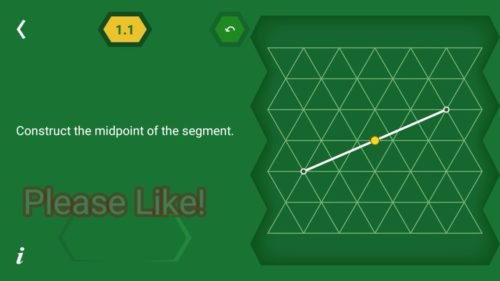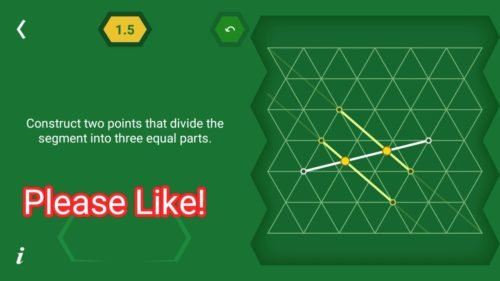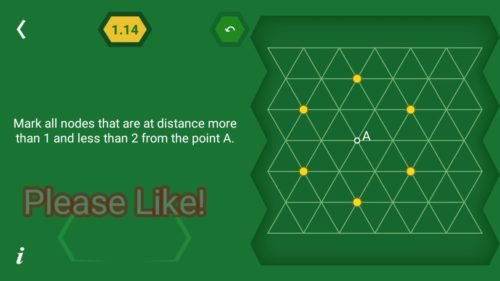# Pythagorea 60 degree Level 1.1 1.2 1.3 1.4 1.5 1.6 1.7 1.8 1.9 1.10 1.11 1.12 1.13 1.14 1.15 Solution/Answers

Solutions, Hints, and Answers to pythagorea 60° are available in this post, Scroll down to find solutions to all the levels.

Pythagorea sixty degrees is android/iOS app developed by Horis International Limited. This game is mostly focused on geometric puzzles and construction. The workspace is divided into triangular grids lines aligned 60° to each other, that’s why this game names as pythagorea 60°. You should know all the basic Math operations. All lines and shapes are drawn on a grid whose cells are equilateral triangles. Most of the game levels can be answered using natural intuition and bye some basic laws of geometry.

You have to connect points on the grid using straight lines to construct an element, you can even use intersection points to draw. some levels are very easy some are of medium difficulty and some are very hard to solve, that’s why I am providing solutions to all the problems.

If you are here for levels other than ‘Length and Distance’ Go to directory of all other levels at : http://www.puzzlegamemaster.com/pythagorea-60-complete/

• Pythagorea 60° Level 1.1: Construct the midpoint of the segment.• Pythagorea 60° Level 1.2: Construct a line through the point A and the midpoint of the segment.• Pythagorea 60° Level 1.3: construct the midpoint of the segment.• Pythagorea 60° Level 1.4: Construct two points that divide the Segment into three equal parts.• Pythagorea 60° Level 1.5: Construct two points that divide the Segment into three equal parts.• Pythagorea 60° Level 1.6: Select all nodes at distance 2 from the point A.• Pythagorea 60° Level 1.7: Mark a node that is at equal distance from the three points.• Pythagorea 60° Level 1.8: Construct a point that divides the polyline into two parts of equal length.• Pythagorea 60° Level 1.9: Mark a node that is at equal distance from the three points.• Pythagorea 60° Level 1.10: Construct a point that divides the polyline into two parts of equal length.• Pythagorea 60° Level 1.11: Mark a node that is at equal distance from the three points.• Pythagorea 60° Level 1.12: Construct a point that divides the polyline into two parts of equal length.• Pythagorea 60° Level 1.13: Construct the midpoint of the segment.• Pythagorea 60° Level 1.14: Mark all nodes that are at distance more than 1 and less than 2 from the point A.• Pythagorea 60° Level 1.15: Mark all nodes that are at equal distance from the two given points.Feel free to comment below if you have any doubts regarding the solutions. I will try to help you guys. All other levels are posted on this blog please visit them too.